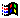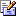Contents Articles Behaviors Books Director News Director Web Sites FAQ Games Mailing Lists News Groups Project Examples Reviews Software Tools Useful Web Sites Utilities Xtras

 Don't miss theseSaving Global Variables to a File

 Compatibilities:This item has not yet been rated

Author: MediaMacros (website)

I have a game that needs to save out all of my global variables (item lists, location, etc.) to a save file. Is there an easy way to do this?

 The basic way to handle this would be to use the FileIO Xtra to save out a text file of each variables value, then write a handler to check a specific line or section of the file to import into a specific variable. Something like this... set myValue1 = line 1 of the text set myValue2 = line 2 of the text etc. With Director 7, it can be a bit easier. Using Director 7's the globals list instance we can read and write values to a global without hard coding each one individually. If you type... put the globals into the message window you get a result of (the globals). Not too helpful, but if you discover that you can find a value in that list and reference it as a property list, then it becomes much more powerful... test = "Test me" put test -- "Test me" put the globals[#test] -- "Test me" As you can see, you can reference each member in the globals list as a property or as the variable. From this we can easily isolate each variable's name using the getPropAt command and then get its type (ilk) and value. Before we begin coding we need to look at how we write to the file. We will need to know the type of variable, it's name and the content. We can't count on each line being a separate entry, since text strings can span multiple lines, so we need to have something to distinguish which lines are a break between variables, which are variable names, which are the variable types, and all else will be the variable's content. Using this as the basic content here is the script I came up with... on saveGlobalList theFileName --set a variable delimiter varDel = "New Variable Line Starts Here" globalFile = "" --create the content of the file repeat with x = 1 to (the globals).count globalFile = globalFile & varDel & ¬ return & "Value Type = " & (the globals)[x].ilk &¬ return & "Value Name = " & string(getPropAt(the globals, x)) &¬ return & (the globals)[x] & return if x = (the globals).count then globalFile = globalFile & varDel end if end repeat --write the file myFile = new(xtra "fileio") if fileExists(theFilename) = 0 then deleteFile(theFilename) end if createFile(myFile, theFilename) openFile(myFile, theFilename, 2) writeString(myFile, globalFile) closeFile(myFile) myFile = 0 end on loadGlobals theFileName --get the file contents myFile = new(xtra "fileio") if fileExists(theFilename) = 0 then openFile(myFile, theFileName, 1) fileContents = readFile(myFile) closeFile(myFile) myFile = 0 else alert "File does not exist" end if valType = "Value Type = " valName = "Value Name = " theType = "string" theName = "" theValue = "" varDel = "New Variable Line Starts Here" repeat with x = 1 to fileContents.line.count --check to see if you are starting a new variable and if so write the value to the variable if fileContents.line[x] starts varDel and theName <> "" then if theType = "string" then (the globals)[symbol(theName)] = string(theValue) else (the globals)[symbol(theName)] = value(theValue) end if theType = "string" theName = "" theValue = "" else if fileContents.line[x] starts varDel and theName = "" then --allows line 1 to write correctly nothing else if fileContents.line[x] starts valType then theType = (fileContents.line[x]).char[(valType.char.count + 1)..(fileContents.line[x].char.count)] else if fileContents.line[x] starts valName then theName = (fileContents.line[x]).char[(valName.char.count + 1)..(fileContents.line[x].char.count)] else if theValue = "" then theValue = fileContents.line[x] else theValue = theValue & return & fileContents.line[x] end if end if end if end repeat end on getBehaviorDescription me return = "This handler saves a test file with all global variables and can then re-import them. This makes use of lingo's -the globals- variable to get and set the global variables." end This will write out a file (using FileIO) that has the line "New Variable Line Starts Here" to break each variable off from the previous one. It passes the type of value so that "3" will not end up being set to the number 3, etc. This is certainly not the only way to do this. Using an Xtra like Buddy API you can write a file out that is set up in a variable = value format and even encrypt the data so it can't be tampered with. TO flesh it out more you can also add error checking, checking to make the file "hack proof", etc. but this will get the basic job done.

 Contact MMI 36 South Court Sq Suite 300 Newnan, GA 30263 USASend e-mail